Academics
Departments and Curriculum

# Mathematics

The teaching of mathematics at Holderness is founded on a practice of collaboration that deepens understanding in two ways. First, we believe that working in partnership with others provides an important exercise in articulating mathematical thinking. Our students read or hear new concepts and then discuss this knowledge with their peers—a process that fosters both active and passive learning, as well as accountability. Second, in working collaboratively, our students develop their critical listening skills. As they seek to understand their peer collaborators, they develop openness and flexibility with regard to their mathematical analyses.

### Values

Connections: Students are exposed not only to the many connections present within mathematics as a discipline, but also the exciting connections that occur between mathematics, other fields of study, and a variety of possible career paths. Our students use current events, data from websites and companies, and other available sources to apply classroom concepts to real world applications.

Collaboration: Holderness students work in collaborative learning environments so as to deepen their understanding of mathematical concepts, allowing students the opportunity to articulate their own thinking and to develop the listening skills that allow them to enrich their understanding through teamwork.

Technology: Students have the opportunity to learn and review concepts by using flipped classrooms created by teachers, video tutorials, and Smartboard classroom notes. Students are also able to hone problem solving skills by completing online practice problems. Technology, including graphing calculators and specialized software (e.g., Excel and Fathom), are introduced and explored as important tools to be used in the study of mathematics. We recognize that technology is an important tool; however we also believe that core skills and mental math are important.

Develop Independent and Critical Thinking: We build math confidence. We develop a work ethic towards math. Through problem solving techniques, utilization of extra help, nightly homework, and group projects, our students learn to be confident mathematicians who can think creatively. We recognize that we are preparing our students for jobs and a world that is currently unknown to us and to them, and our goal is for them to be curious and able to adapt to difficult problems.Click here for more student work photos

• ## Algebra 1

This is a cornerstone course designed to prepare students to investigate and master more complex, but related, concepts studied over the balance of the mathematics curriculum. Students review the language of algebra, real number operations, and approaches used to solve linear equations before engaging in an expanded study of function graphing, linear analysis, methods of solving linear inequalities, and systems of both inequalities and linear equations. Multiplication of polynomials, factoring, and solving quadratic and exponential functions, together with simplification and evaluation of radical expressions, constitute the remainder of the course.

##### Order Your Textbook(s)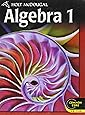A TI-84 Plus calculator is required.
Click on the book(s) above for direct purchasing options. Please note: if you would like to find the books through another vendor, be sure to take note of the exact ISBN numbers so you/your child has the correct book for class.
• ## Geometry

This course covers the fundamental concepts of geometry, with algebra and discrete mathematical topics woven into the curriculum. Topics include reasoning and proof, building blocks of geometric shapes, congruence and similarity, right triangles and trigonometry, transformations of figures and equations, and surface area and volume.

##### Order Your Textbook(s)
A TI-84 Plus calculator is required for this class. No textbook required.
• ## Algebra 2

In this course, students continue the study of concepts introduced in Algebra I and Geometry. The concept of function is introduced and families of functions are introduced as important tools to explore, analyze, and make conjectures as we further develop mathematical problem-solving skills. Using real-world examples, students explore quadratic equations, polynomials, and operations of functions. Students also examine properties, applications, and graphs of absolute value, exponential, logarithmic, power, and radical functions.

##### Order Your Textbook(s)
A TI-84 Plus calculator is required. No textbook required.
• ## Honors Algebra 2

In this honors course, students continue the study of concepts introduced in Algebra I and Geometry. Concepts such as linear functions, linear regression, data analysis, matrices, and systems of equations are introduced as important tools to explore, analyze, and make conjectures as we further develop mathematical problem-solving skills. Using real-world examples, students explore quadratic equations, polynomials, and operations of functions. Students also examine properties, applications, and graphs of absolute value, exponential, logarithmic, power, radical, and trigonometric functions.

##### Order Your Textbook(s)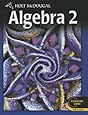A TI-84 Plus calculator is required.
*Students: Please email Ms. Wolf (ewolf@holderness.org) before you buy this book; she has several copies available and would be happy to have you borrow one for the year.

Click on the book(s) above for direct purchasing options. Please note: if you would like to find the books through another vendor, be sure to take note of the exact ISBN numbers so you/your child has the correct book for class.
• ## Precalculus

This course offers a more rigorous approach to the study of functions and to the topics needed for calculus. The emphasis of this course is on analysis through formal mathematical notation and the relation of mathematical concepts to real world scenarios. Major topics include linear, exponential, and logarithmic functions; trigonometric functions; polynomials; radical functions; mathematical limits; composition of functions; and inverses of functions.

##### Order Your Textbook(s)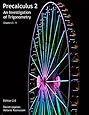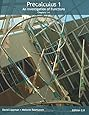A TI-84 Plus calculator is required.
If you only want an electronic copy, you may access the free text here: opentextbookstore.com/precalc/
Click on the book(s) above for direct purchasing options. Please note: if you would like to find the books through another vendor, be sure to take note of the exact ISBN numbers so you/your child has the correct book for class.
• ## Computer Science

Python is an accessible programming language whose simple yet powerful structure and easy-to-use development environment allow students to achieve impressive results quickly. Using this programming language, students will learn the basics of Computer Science including variables, conditional statements (if-else), iterations (loops) and the fundamentals of programming design and implementation. We will also utilize Python’s ‘turtle graphics’ module, which allows for two-dimensional designs. Throughout the course, we will discuss the challenges and opportunities related to the explosion of computer use in the modern world.

##### Order Your Textbook(s)
No textbook required.
• ## STEM Robotics I

As an introductory course in robotics the students will learn JAVA computer programming as well as problem solving strategies. This course will involve students in the development, building and programming of a Tetrix robot. Working in teams they design, build, program and document their progress. Topics include motor control, gear ratios, torque, friction, sensors, timing, program loops, logic gates, decision-making, timing sequences, propulsion systems and binary number systems. Student designed robots will be programmed to compete in a variety of autonomous and remote controlled challenges.

##### Order Your Textbook(s)
No textbook required.
• ## Honors Precalculus

This course is designed for students who have completed Honors Algebra 2. Honors Precalculus covers all of the topics studied in the regular pre-calculus course; however, the pace is slightly faster than regular pre-calculus so that the students will be able to cover more trigonometry and topics that will prepare them for AP Calculus. By the end of the class the students will not only be introduced to topics that are vital to understanding Calculus, such as continuity and limits, but they will also be introduced to the derivative through the limit definition.

##### Order Your Textbook(s)A TI-84 Plus calculator is required.
If you only want an electronic copy, you may access the free text here: opentextbookstore.com/precalc/
Click on the book(s) above for direct purchasing options. Please note: if you would like to find the books through another vendor, be sure to take note of the exact ISBN numbers so you/your child has the correct book for class.
• ## Advanced Precalculus

This course is designed for students who have done very well in Algebra II. Advanced Precalculus covers all of the topics studied in the regular pre-calculus course; however, the pace is slightly faster than regular pre-calculus so that the students will be able to cover more trigonometry and topics that will prepare them for AP Calculus. By the end of the class the students will not only be introduced to topics that are vital to understanding Calculus, such as continuity and limits, but they will also be introduced to the derivative through the limit definition.

##### Order Your Textbook(s)
A TI-84 Plus calculator is required. No textbook required.
• ## Topics in Mathematics

In this year-long course, students study mathematics in contexts and develop their abilities to problem solve, reason, and communicate.  Real world situations provide the contexts for mathematical investigation.  The topics include but are not limited to: logic, numeration, statistics, financial mathematics, history of mathematics, and functions.

##### Order Your Textbook(s)
Please have a TI-84 Plus calculator. No textbook required.
• ## STEM Robotics II

The STEM Robotics II course builds upon the skills students learned in STEM Robotics I. Working in teams, students will design, build, and program Tetrix robots which must complete more complex and more precise tasks with less margin for error than the projects in STEM Robotics I.

##### Order Your Textbook(s)
No textbook required.
• ## Calculus

Calculus begins with a thorough review of standard high school mathematics in preparation for Calculus and the SAT. The class is designed to introduce students to important Calculus topics and to prepare them for college Calculus. The course will cover the conceptual basis of Calculus including the limit definition of the derivative and Riemann Sums. It will also cover in great detail the rules for differentiation and introduce the integral. By the end of the class students will be exposed to the applications of Calculus with Related Rate and Optimization problems.

##### Order Your Textbook(s)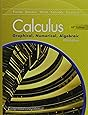Please also have a TI-84 Plus calculator.
Click on the book(s) above for direct purchasing options. Please note: if you would like to find the books through another vendor, be sure to take note of the exact ISBN numbers so you/your child has the correct book for class.
• ## AP Calculus AB

AP Calculus is primarily concerned with developing a student’s understanding of the concepts of calculus through the use of the unifying themes of derivatives, integrals, limits, approximation, and applications and modeling. The course emphasizes a multi-representational approach to calculus, with concepts, results, and problems being expressed graphically, numerically, analytically, and verbally. Students are expected to take the AP exam in May.

##### Order Your Textbook(s)Please also have a TI-84 Plus calculator.
Click on the book(s) above for direct purchasing options. Please note: if you would like to find the books through another vendor, be sure to take note of the exact ISBN numbers so you/your child has the correct book for class.
• ## AP Statistics

This is the high school equivalent of a one-semester, introductory college statistics course. In this course, students develop strategies for collecting, organizing, analyzing, and drawing conclusions from data, with a focus on univariate and bivariate data. Simulations and probability aid students in constructing models for chance phenomena and lead to the study of inference, where students learn to complete confidence intervals and hypotheses tests. Students use technology, such as the Ti calculator, Fathom, and online applets, to strengthen their understanding of statistical concepts. Throughout the year, students apply statistical skills learned in class to analyze data from a variety of sources, including data from our athletic teams, student-generated data, and online sources. The coursework culminate with AP exam in May.

##### Order Your Textbook(s)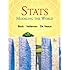Please also have a TI-84 Plus calculator.
Click on the book(s) above for direct purchasing options. Please note: if you would like to find the books through another vendor, be sure to take note of the exact ISBN numbers so you/your child has the correct book for class.
• ## AP Computer Science

This year-long course introduces students to computer science with fundamental topics that include problem solving, design strategies and methodologies, organization of data (data structures), approaches to processing data(algorithms), analysis of potential solutions, and the ethical and social implications of computing. The course emphasizes both object-oriented and imperative problem solving and design. These techniques represent proven approaches for developing solutions that can scale up from small, simple problems to large, complex problems. The course is designed For 11th and 1 zth grade students who have completed Algebra 2 with a grade of B or higher.

##### Order Your Textbook(s)
Please have a TI-84 Plus calculator. No textbook required.
• ## AP Economics

Course description coming soon. This course counts as a History course and is staffed by the Math department.

##### Order Your Textbook(s)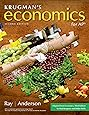Please also have a TI-84 Plus calculator.
Click on the book(s) above for direct purchasing options. Please note: if you would like to find the books through another vendor, be sure to take note of the exact ISBN numbers so you/your child has the correct book for class.
• ## AP Calculus BC (semester)

AP Calculus BC is a semester course designed for students who have completed AP Calculus AB. The course is a continuation of Calculus AB and emphasizes a multi-representational approach to calculus, with concepts, results, and problems being expressed graphically, numerically, analytically, and verbally. The course will cover advanced integration, differential equations, sequences and series, (including Taylor Series and Maclaurin Series), polar functions, and parametric functions. Students are expected to take the AP Calculus BC exam in May.

##### Order Your Textbook(s)Please also have a TI-84 Plus calculator.
Click on the book(s) above for direct purchasing options. Please note: if you would like to find the books through another vendor, be sure to take note of the exact ISBN numbers so you/your child has the correct book for class.
• ## AP Calculus BC (full-year)

Pre-requisite: Advanced Precalculus at Holderness or permission by the department chair. AP Calculus is primarily concerned with developing a student’s understanding of the concepts of calculus through the use of the unifying themes of derivatives, integrals, limits, approximation, and applications and modeling. The course emphasizes a multi-representational approach to calculus, with concepts, results, and problems being expressed graphically, numerically, analytically, and verbally. Students are expected to take the AP exam in May.

##### Order Your Textbook(s)Please also have a TI-84 Plus calculator.
Click on the book(s) above for direct purchasing options. Please note: if you would like to find the books through another vendor, be sure to take note of the exact ISBN numbers so you/your child has the correct book for class.
• ## Differential Equations

Differential Equations is a semester-long course for students who have completed AP Calculus AB and/or BC. Students will study ways to solve differential equations graphically, numerically, and algebraically. Students will also study the real world applications of these types of equations.

##### Order Your Textbook(s)
More information coming soon.
• ## Linear Algebra

Not offered in 2021-22

Linear Algebra is a semester course for students who have completed AP Calculus AB and/or BC. There are widespread applications of Linear Algebra to business, engineering, and higher level mathematics. The main topics covered are systems of linear equations, matrices, Gaussian elimination, Gram-Schmidt orthogonalization, determinants, vector spaces, eigenvalues, and eigenvectors. Students explore a wide range of applications and may use computer software.

##### Order Your Textbook(s)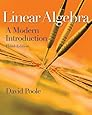Please also have a TI-84 Plus calculator.
Click on the book(s) above for direct purchasing options. Please note: if you would like to find the books through another vendor, be sure to take note of the exact ISBN numbers so you/your child has the correct book for class.

# Faculty

•Elizabeth Wolf
Mathematics Department Chair
(603) 779-5343
St. Paul's School
University of Pennsylvania - BA Mathematics
University of New Hampshire - MST Mathematics
•Joseph Arsenault
Mathematics Faculty
(603) 779-5349
•Ian Casey
Mathematics Faculty
(603) 779-5356
Buckingham Browne and Nichols School
Wheaton College - B.A. Mathematics
•Leigh Anne Connors
Mathematics Faculty
(603) 779-5348
Northwood School
Plymouth State University - Mathematics
•John Donovan
Math Teacher
(603) 779-5321
SUNY (Buffalo) - PhD
Hartwick College (NY) - BA
Harborfields high School
SUNY Buffalo - MA
Hauppauge High School
Hartwick College
•Richard Eccleston
Athletics Director & Mathematics Faculty
(603) 779-5371
Holderness School
Hobart College - BA
•Duane Ford
Mathematics Faculty & Director of Job Programs
(603) 779-5300
Middlebury College (VT) - BA
Holderness School (NH)
Columbia University (NY) - MA
•Pam Mulcahy
Mathematics Faculty
(603) 779-5332
University of New Hampshire - MEd
Harwich High School
Smith College - BA
•Morgan Stepp
STEM Faculty
(603) 779-5299
•Victoria Stigum
Mathematics Faculty
(603) 779-5312
Lafayette College (PA) - BA
Choate Rosemary Hall
University of New Hampshire - M.B.A.

## Take The NextStep

Is Holderness right for you?  The best way to find the right boarding school is to reach out. Check us out in person in New Hampshire or virtually.  See why Holderness is more than a school, it's an elevated experience.
Holderness School
33 Chapel Lane, Holderness, NH 03245

MAIL P.O. Box 1879 Plymouth, NH 03264-1879
PHONE (603) 536-1257
EMAIL info@holderness.org